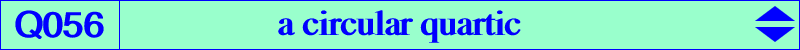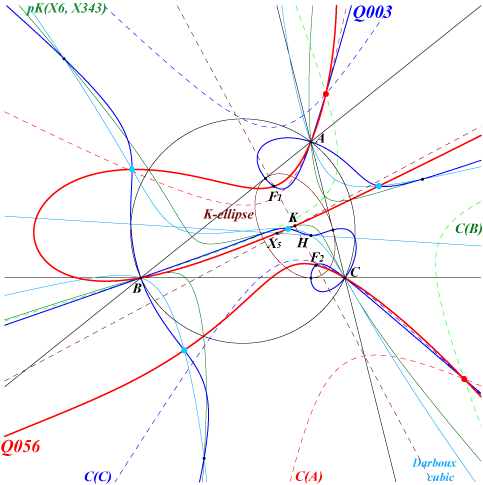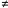too complicated to be written here. Click on the link to download a text file.X(5), X(6), X(2574), X(2575) CPCC or H-cevian points, see Table 11 Ix-anticevian points, see Table 23 isogonal conjugates of the Ix-anticevian points, see Table 23 foci of the K-ellipse (inellipse with center K when the triangle ABC is acute angle) common points of C(O,R) and pK(X6, X343).Q056 is a circular quartic with singular focus X(858), the complement of X(23). It has two real asymptotes parallel to those of the Jerabek hyperbola. Q056 contains the CPCC points (light blue points on the figure) and the Ix-anticevian points (red points). See also Q003, the Euler-Morley quintic. Indeed, if we consider the conic C(A) in table 23 and the conic Ca in table 11, then, for a given real t, the conic CtA = (1-t) C(A) + t Ca is a conic from the pencil generated by the two former ones. Similarly define CtB and CtC. These three conics CtA, CtB, CtC belong to a same pencil F(t) for any t hence they must have four common points not neccessarily all real. As t varies, the locus of these common points is Q056. When t = 0, we find the Ix-anticevian points again and, when t = 1, we find the CPCC or H-cevian points again. When t = -1/3, all the conics of F(t) are rectangular hyperbolas. When t-1/3, F(t) contains one and only one rectangular hyperbola H(t) passing through X(5), X(6), X(2574), X(2575). It degenerates into two perpendicular lines for three values of t. One of them is t = 1/3 in which case the conics CtA, CtB, CtC are circles. The remaining two are rather complicated. When F(t) contains X(6), it also contains the points of Q056 on the circumcircle. These points lie on the rectangular hyperbola H(t) passing through X(22), X(110), X(343), X(2917).